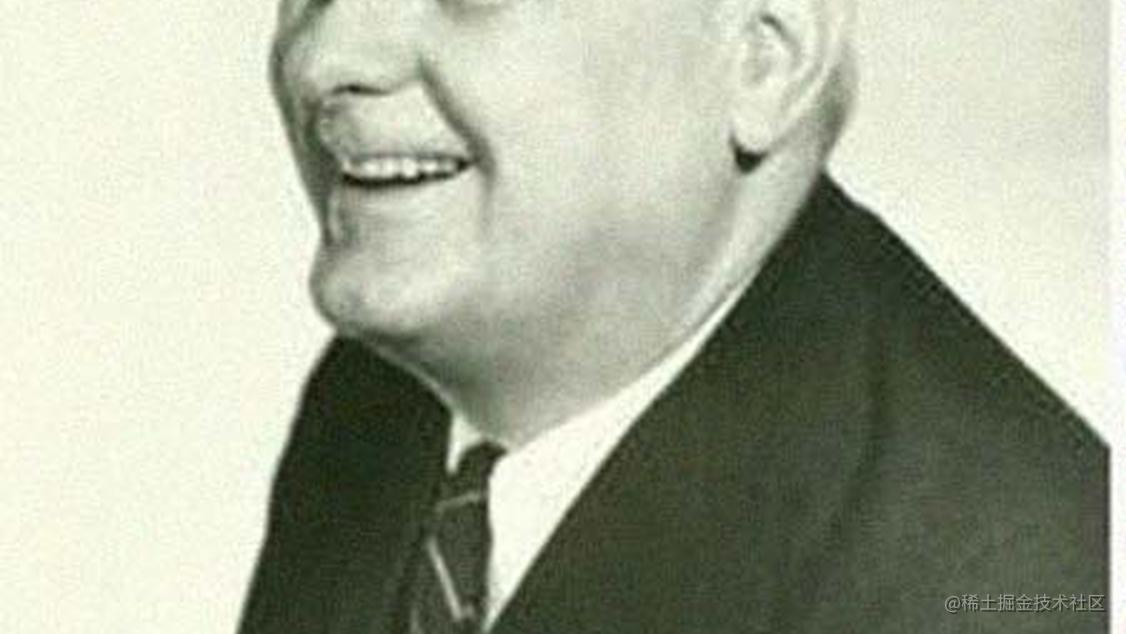# 讲给产品同学的λ演算入门课λ是希腊字母表的第11个字母，大写为Λ，英语名称为Lambda，中文读音“兰木达”。既然是谈演算，总得有数字吧，所以下面我们先来回顾一下什么是数字。

0λxy.y
1λxy.x(y)
2λxy.x(x(y))
3λxy.x(x(x(y)))

# 2 什么是函数

f(x)=x+1就是一个函数，而且是个一元函数。我们把等号左边的f叫做函数名，括号里的x叫做自变量，等号右边的x+1叫做函数体或函数表达式。等号左边的整体f(x)叫做函数签名，签名包含了函数名和参数。

`f(x)=x+1，表示为：λx.x+1 ； f(x,y)=x+y，表示为：λxy.x+y`

# 3 进行λ演算

`λxyz.y(xyz)，对x代入a，结果是：λyz.y(ayz)`

`λxyz.y(xyz)，对x,y分别代入a,b，结果是：λz.b(abz)`

`λxyz.y(xyz)，对x,y,z分别代入a,b,c，结果是：b(abc)`

`λxyz.y(xyz)，对x代入(λab.b)，得到：λyz.y((λab.b)yz)。`

`1P2`
`= (λab.a(b)) (λxyz.y(xyz)) (λmn.m(m(n))) //为了避免歧义这里加了3对括号`
`= λb.(λxyz.y(xyz))(b) (λmn.m(m(n))) // 第1步演算，用P代替1中的a变量`
`= (λxyz.y(xyz)) (λmn.m(m(n))) // 第2步演算，用λmn.m(m(n))代替b变量`
`= λyz.y((λmn.m(m(n)))yz) // 第3步演算，用λmn.m(m(n))代替x`
`= λyz.y(y(y(z)) // 第4步演算，用y代替m、z代替n`
`= 3 `

# 4 总结

λ演算是一套形式化系统、一种计算模型，由数学家阿隆佐·邱奇在20世纪30年代首次发表。λ演算可以清晰地定义什么是一个可计算函数，任何可计算函数都能以这种形式表达和求值，它可以完全替代单一磁带图灵机。

λ演算的几个核心概念：
①变量：  写在符号λ和点(.)之间的字母。
②函数：  也叫抽象，即由符号λ、变量、点以及其后的表达式构成。
③应用：  由一个函数跟上要替换变量的参数构成，例如`λab.a(b) C`就是一个应用，其含义是将函数`λab.a(b)`应用于参数C，此应用的结果是`λb.C(b)`
④λ项：  对变量、函数、应用的统称，λ项是组成一行λ表达式的构成部分。